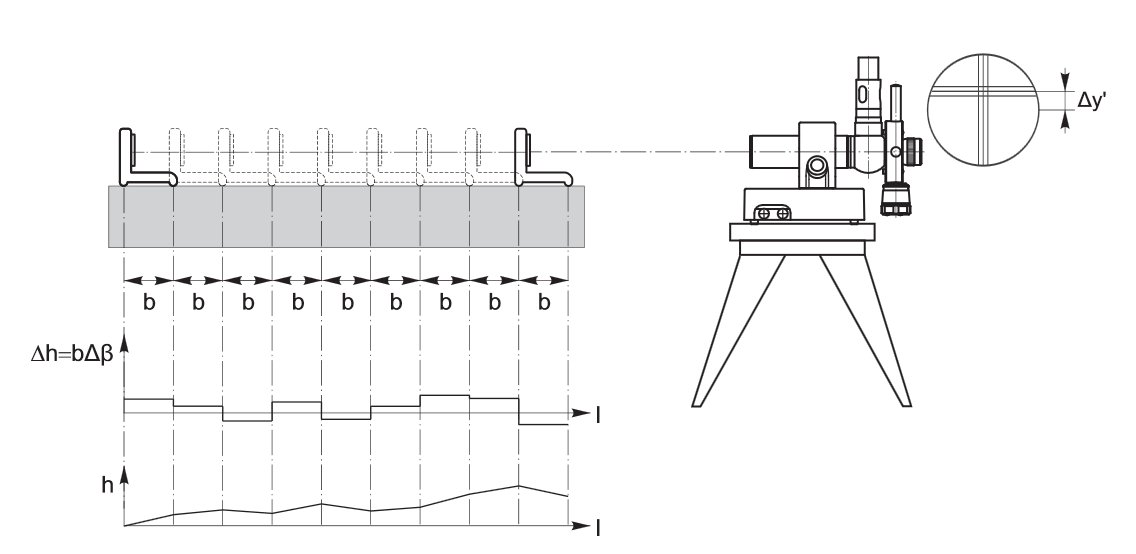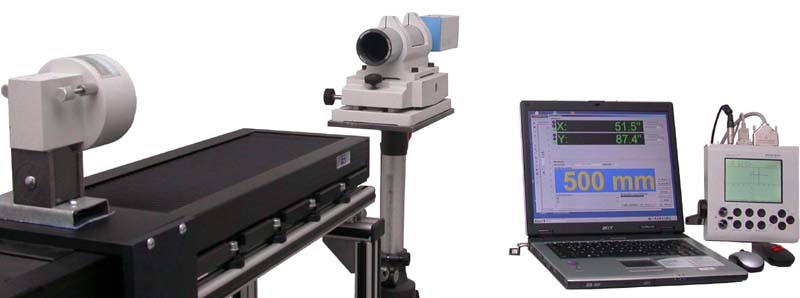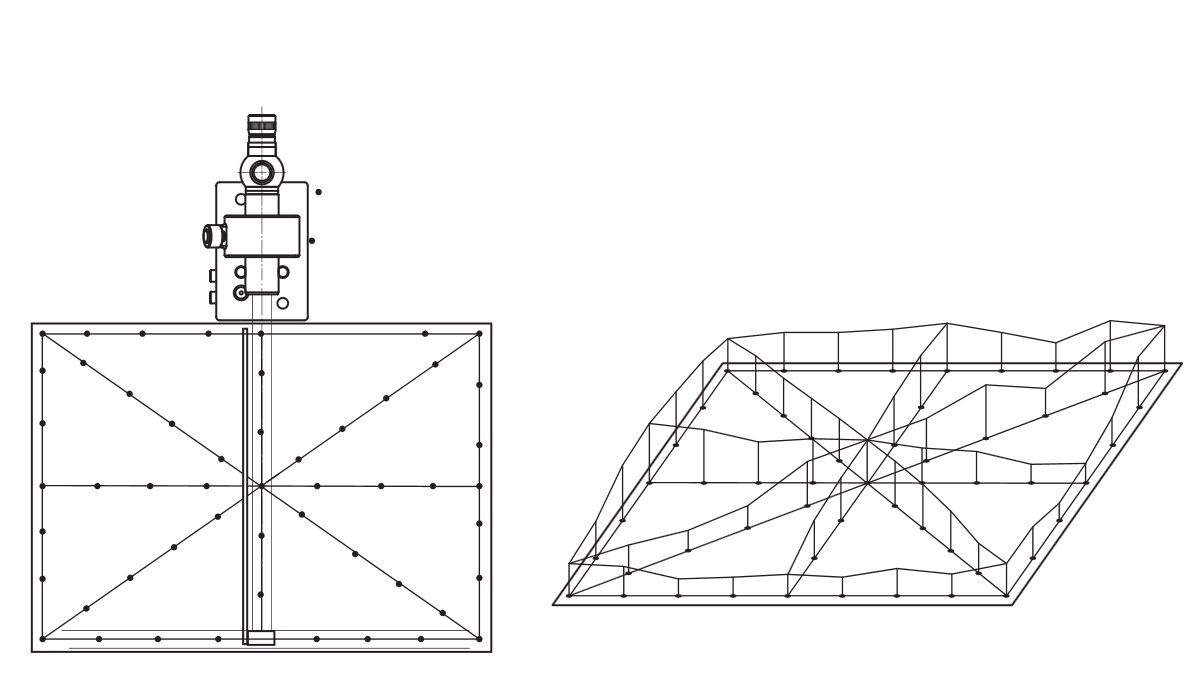Optical metrology · Software development · Image processing

Optical metrology · Software development · Image processing

• Welcome

• Company

• News

• Exhibitions

• Products

• Optics Test Equipment

• Meters for Contact angle and surface tension

• measuring systems for beverage can manufacture

• drawing die and wire measurement

• Measurement of Straightness, Flatness, positioning uncertainty of round tables

• Semiconductor technology, Micro scriber

• Measuring microscopes, Image processing

• Flatness, Bow, Warp, curvature, glass thickness

• Measuring systems for night vision systems

• Product videos

• COMEF

• ELCOLEVEL

• ELCOWIN

• RTM

• SURFTENS

• Teamviewer

• Contact

• ISO 9001 Certificate

• Representatives

Products > Measurement of Straightness, Flatness, positioning uncertainty of round tables > ELCOWIN - Software for 2D-straightness measurement with electronic autocollimators

The software ELCOWIN serves for the measurement of straightness, squareness, parallelism of slides and flatness of precision tables by the autocollimation principle. ELCOWIN can be used in connection with electronic autocollimators of the ELCOMAT series from Moeller-Wedel-Optical.

Get in touch >

The autocollimation principle

The autocollimator projects the image of a reticle pattern in collimated beam path to infinity. A mirror in the optical path reflects the beam back into the autocollimator.

The reflected beam generates the autocollimation image.

When the mirror is perpendicular to the optical axis, the beam is reflected into itself.

When the mirror is tilted with an angle alpha , the incident angle of the beam on the mirror is epsilon = alpha . After reflection the beam enters the objective obliquely with an angle of  delta = 2*Epsilon. Depending on the angle of the ray bundle to the optical axis, the autocollimation image is shifted from the centre.

The shift of the autocollimation image from the centre in both x0 and y0 directions is a measure for the tilt of the mirror.

Autocollimators that are calibrated to tilting angle show half the angle of the reflected beam to the optical axis on their reading and therefore directly the tilting angle of the mirror.

Autocollimation-Examples for applicationPrinciple of straightness measurement by autocollimation principlePractical setup for straightness measurement of a slide by autocollimation principleStraightness measurement

The straightness measurement is the plase dependent measurement of the tilting angle of the reference mirror.

The reference mirror is set on slide. The autocollimator is adjusted to the mirror to zero position in x and y.

For the measurement, the slide with the reference mirror is moved in defined steps.

Each tilting of the mirror in the guiding direction effects a displacement of the autocollimation image from the zero-position. The displacement in each point is measurement.

ELCOWIN calculates from the displacement data the form of the slide.

Flatness measurementFlatness measurement with autocollimation principleFor the measurement of planarity of surfaces several straightness measurements (s. p. 30) are carried out. The straightness measurements form a geometrical pattern, e.g. the so-called »Union Jack« pattern (fig. a). The autocollimator is

put at one end of the line of measurement and adjusted such, that the light beam from the autocollimator is propagating in the direction of measurement.

A straightness measurement is carried out using the base mirror. A ruler can be used as a guide way for the base mirror. This procedure is repeated for the other lines of measurement.

The height profiles of the lines are combined to a two dimensional pattern, here the »Union Jack«, by linking at the intersection points (fig. b).

This evaluation is most easily carried out on a computer, using the special software ELCOWIN.

With the use of the ELCOMAT3000 or ELCOMAT vario the accuracy can be increased with an even shorter measurement time.

In this case the time-consuming manual data input is reduced.